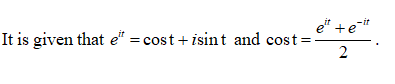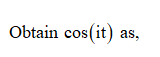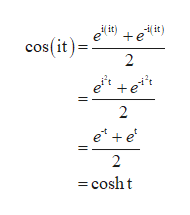# From Euler’s formula e^it= cos(t) + isin(t) show thata) cos(t)= 1/2(e^it+e^-it) then show cos(it)=cosh(t)= 1/2(e^t+e^-t) and cosh(it)= cos(t)

Question
9 views

From Euler’s formula e^it= cos(t) + isin(t) show that

a) cos(t)= 1/2(e^it+e^-it) then show cos(it)=cosh(t)= 1/2(e^t+e^-t) and cosh(it)= cos(t)

check_circle

Step 1Step 2...help_outlineImage Transcriptionclosei( it) i it) +e cos(it)= e +e** e* + e 2 = cosht fullscreen

### Want to see the full answer?

See Solution

#### Want to see this answer and more?

Solutions are written by subject experts who are available 24/7. Questions are typically answered within 1 hour.*

See Solution
*Response times may vary by subject and question.
Tagged in

### Math